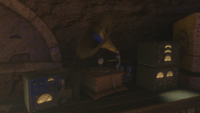• # Song LyricsWhere Are We Going?

Where are we going

from here

Where do we go

Are we all blinded

by fear

how do we know

how do we know

Where do we go

Where do we go

How do we know

Where do we go

Where are we going

from here

How do we know

Where do we go

(In the lyrics video: taT GgA ccT cag aGa cca cGg att gaC gaa Tca acc)

Where are we going

from here

Do we let go of all we know

Are we all blinded

by fear

Where do we go when we let go

I feel I'm falling

from here

Don't let me go

Is it the calling

we hear

we hear

how do

how do

we know

## User Feedback

There are no comments to display.

×

• Story